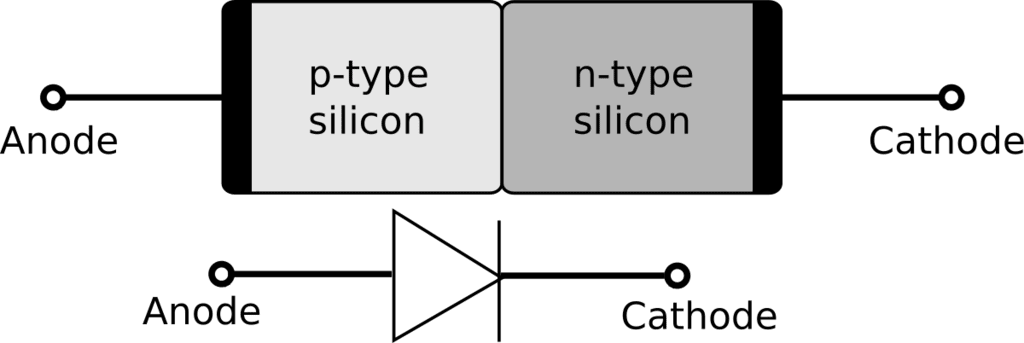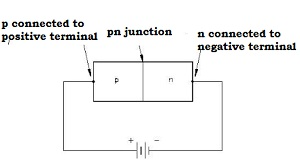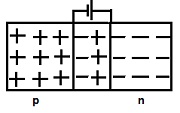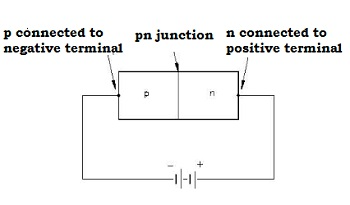Courses

# P-n Junction Diode (Forward & Reverse Bias) Class 12 Notes | EduRev

## JEE : P-n Junction Diode (Forward & Reverse Bias) Class 12 Notes | EduRev

The document P-n Junction Diode (Forward & Reverse Bias) Class 12 Notes | EduRev is a part of the JEE Course Physics For JEE.
All you need of JEE at this link: JEE

p-n Junction Diode

• A semiconductor diode is a p-n junction with metallic contacts provided at the ends for the application of an external voltage
• Thus p-n junction diode is a two terminal device represented as• The equilibrium potential barrier can be altered by applying an external voltage V across the diode

There are two methods of biasing a p-n unction – Forward bias and reverse bias

Forward biasing

• If the positive terminal of the external battery is connected to the p-side and the negative terminal of the external battery is connected to the n-side, then the p-n junction is said to be forward biased• The direction of the applied voltage V is in a direction opposite to that of the potential barrier setup at the junction• As a result of this, the depletion layer width decreases and the barrier height is reduced. The effective barrier height under forward bias is V– V
• If the applied voltage V is small, the barrier potential will be reduced only slightly below the equilibrium value. Hence, only small number carriers will possess energy to cross the junction. Thus, the current is small
• If the applied voltage V is large, the barrier potential will be reduced significantly. Hence, the current is significant
• Due to the applied voltage, the electrons from the n-side cross the depletion region and reach the p-side. Similarly, the holes from the p-side reach the n-side
• As electrons reach the p-side and electrons are minority carriers in p-region, the forward bias is also known as minority carrier injection
• At the junction, the minority carrier concentration increases significantly
• Due to concentration gradient, the injected electrons on p-side diffuse from the junction edge of p-side to the other end of the p-side
• Similarly, the injected holes on the n-side diffuse from the junction edge of n-side to the other end of n-side
• The motion of charged carriers on either side gives rise to current and is usually measured in mA
• The total diode forward current is sum of hole diffusion current and conventional current due to electron diffusion

Reverse biasing

• If the positive terminal of the external battery is connected to the n-side and the negative terminal of the external battery is connected to the p-side, then the p-n junction is said to be reverse biased• The direction of the applied voltage is same as that of the barrier potential.
• As a result, the barrier height increases and the depletion region widens due to change in electric field.
• The effective barrier height is VB + V.
• This suppresses the flow of electrons from n region to p region and holes from the p region to n region. Hence, diffusion current decreases.
• The electric field direction of the junction is such that if electrons on p-side or holes on n-side in their random motion come close to the junction, they will be swept to its majority zone. This gives rise to drift current of order of few µA.
• The diode reverse current is not much dependent on the applied voltage. Even a small voltage is sufficient to sweep the minority carriers from one side of the junction to the other side of the junction.
• The current under reverse bias is essentially voltage independent up to a critical reverse bias voltage, known as breakdown voltage VBE.
• When V = VBE, the diode reverse current increases sharply. If the current is not limited, the p-n junction will get destroyed.
Offer running on EduRev: Apply code STAYHOME200 to get INR 200 off on our premium plan EduRev Infinity!

## Physics For JEE

187 videos|516 docs|263 tests

,

,

,

,

,

,

,

,

,

,

,

,

,

,

,

,

,

,

,

,

,

;